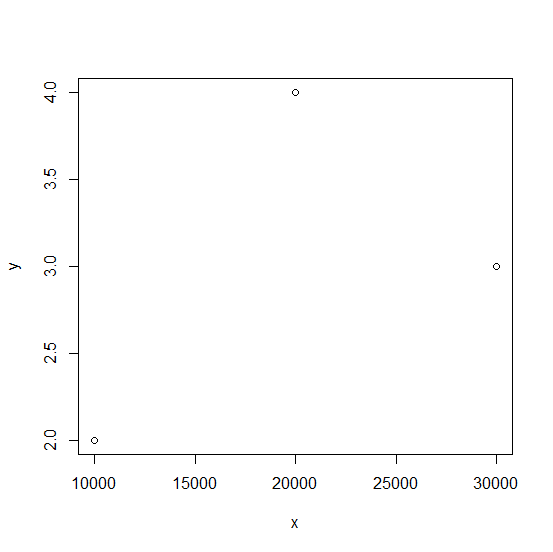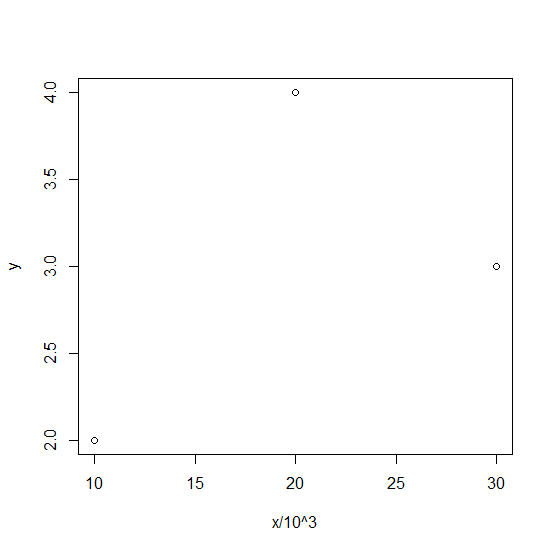# How to display raise to the power on X-axis in base R plot?

To display anything different than the vector or column names on the axes, we need to use xlab for X-axis and ylab for Y-axis. Therefore, if we want to display raise to the power on X-axis then xlab argument will be along with the plot function. For example, if we have a vector called x and we want to create a point chart for x -square with X-axis showing x^2 then it can be done as plot(x^2,xlab="x^2").

## Example

> x<-c(10000,20000,30000)
> y<-rpois(3,2)
> plot(x,y)

## Output## Example

> plot(x/1000,y,xlab="x/10^3")

## Output